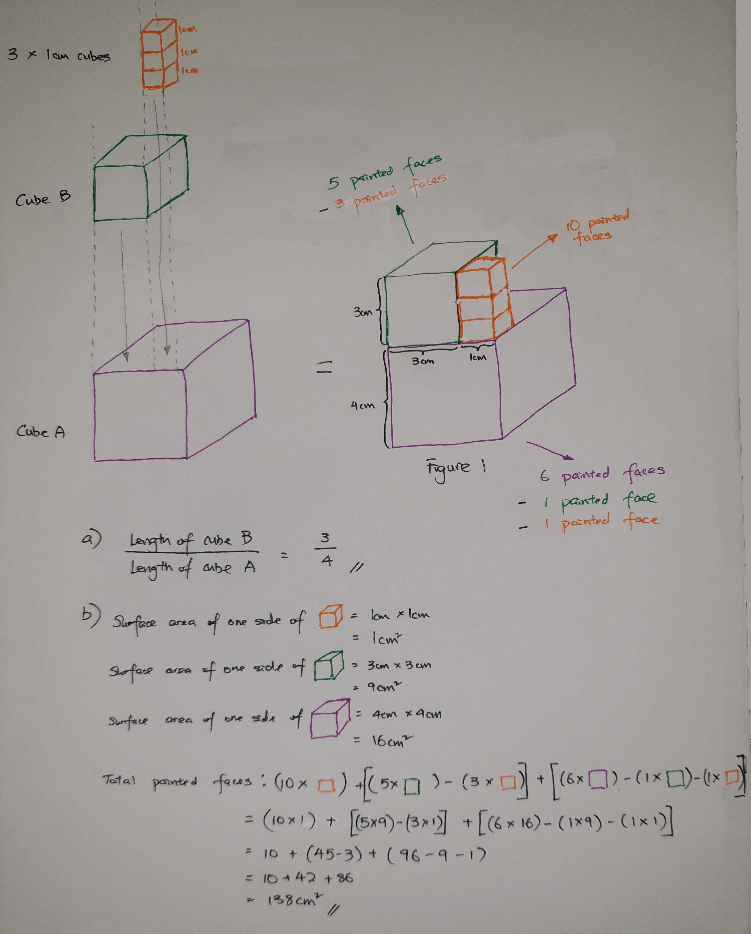# QuestionYou will need some ability to visualise for this question, but I have tried my best to draw it as per attached picture.

a) From picture attached, it should be quite easy to see that the answer is 3/4.

b) I have colour coded the different cubes making up Figure 1 into purple (Cube A), green (Cube B) and orange (1cm cubes) respectively for ease of reference. From picture attached, we can see that the painted surface area of Figure 1, including the base, is made up of:

(10 orange faces) + (5 green faces3 orange faces) + (6 purple faces1 green face1 orange face)
= 10 cm2
+ 42 cm2 + 86 cm2
= 138 cm2

c) Unless I’m reading the question wrongly, I’m not sure there is a clear solution. The height of rectangular Block C is not given, so it is quite impossible to tell how many more 1 cm cubes should be added to make the whole Figure 2 a rectangular cuboid.

If we were to assume Block C is 1 cm taller than Figure 1, then the answer would be 12 cubes (from the back of the green cube and on top of the purple cube) + 6 cubes (behind the orange cubes and on top of the purple cube) + 16 cubes (on top of Block C) = 34 cubes

0 Replies 0 Likes